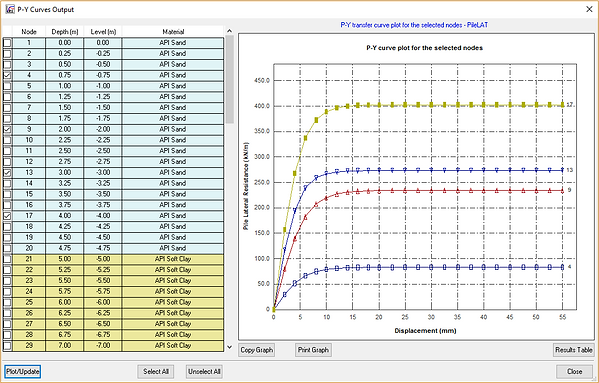## T-Z Curves for Axially Loaded Piles

P-Y curves are the numerical models used to simulate the response of soil resistance (p, soil resistance per unit length of the pile) to the pile deflection (y) for the piles under lateral loading. With this approach, the soils are conveniently represented by a series of nonlinear springs varying with the depth and soil type in the analysis for laterally loaded piles.

This concept was first developed in the 1940’s and 1950’s when energy companies built offshore structures that had to sustain heavy horizontal loads from waves. An exact publication date of the model is not available since the p-y curves are still modified and improved today. The earliest recommendations on p-y curves could be traced back to the 1950s by the works of Skempton and Terzaghi (Ruigrok 2010).

Ideally, p-y curves should be generated from full-scale lateral load tests on instrumented test piles. In the absence of experimentally derived p-y curves, it is possible to use empirical p-y formulations that have been proposed in the literature for different types of soils.

From the 1970s, Further modification and improvement have been given to the p-y method. Instead of giving inputs for the nonlinear spring constant (i.e., the values of the spring constant as a function of pile deflection), p-y curves are given as inputs to the analysis in the p-y method. Different p-y curves have been developed over the years for different soil types, which give the magnitude of soil pressure as a function of the pile deflection.

In the analysis, the pile is divided into small segments, and for each segment, a p-y curve is given as input.  Depending on the magnitude of the deflection of a pile segment, the correct soil resistance is calculated from the p-y curve iteratively.  With the development of the finite element method, analysis using beam finite elements have been widely adopted in many calculations involving the subgrade-reaction approach or the p-y method.  Today, the p-y method is the most widely used method for calculating the response of laterally loaded piles. ​

###### T-Z Curve Models in PileAXL Program

Cohesive Soils

There are five different P-Y curves available for modeling cohesive soils:

1. API Soft Clay. It is recommended in API RP2A 21st Edition (2000). The ultimate resistance of soft clay is determined in the same way as Matlock (1970). The only difference is that the piece-wise curves are used for both static and cyclic loading conditions.

2. Soft Clay (Matlock). This is based on the method established by Matlock (1970) for both static and cyclic loading conditions.

3. Stiff Clay without Water (Reese). This p-y curve model for still clay without water is based on the works by Welch and Reese (1972).

4. Stiff Clay without Water with Initial Modulus. This p-y curve model is based on the works of Welch and Reese (1972) except for the initial slope follows the recommendations on the model of still clay with water by Reese et al. (1975).

5. Stiff Clay with Water (Reese). This p-y curve model is based on the works by Reese et al. (1975) where the lateral ultimate resistance is determined separately for the location near the ground surface and also at deep depth.

Cohesionless Soils

There are three different P-Y curves available for modeling cohesionless soils:

1. API Sand. The p-y curve model follows the recommendations in API RP2A 21st Edition (2000).

2. Reese Sand. The p-y curve model is based on the works of Reese et al. (1974) for both static and cyclic loading conditions.

3. Liquefied Sand. The p-y curve model is based on the works of Rollins et al. (2005).

Rock

There are four different P-Y curves available for modeling rock:

1. Weak Rock - Reese (1997). The p-y curve model is based on the method established by Reese (1997).

2. Strong Rock - Turner (2006). The p-y curve for strong rock is based on the method proposed by Turner (2006).

3. Massive Rock - Liang et al. (2009). The p-y curve for massive rock is based on the method proposed by Liang et al. (2009).

4. Calcareous Rock - Fragio et al. (1985). The p-y curve for calcareous rocks is based on the method proposed by Fragio et al. (1985).

General Geotechnical Materials

There are two options available for modeling the general geotechnical materials:

1. Elastic-Plastic Model. The soil subgrade modulus is determined by Vesic's method and the ultimate lateral resistance is determined by API clay model for cohesive soils, Reese sand model for granular soils and Weak rock - Reese method for rocks.

2. Elastic Model. The elastic subgrade modulus is adopted in the method.

Some p-y curve examples are shown in the figures below.